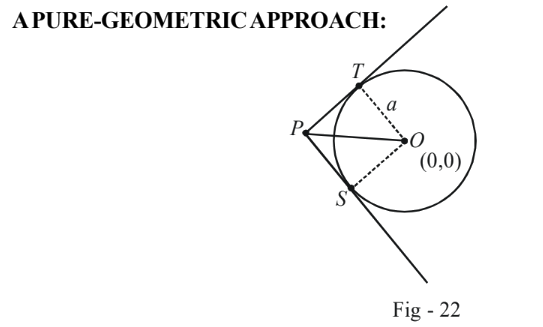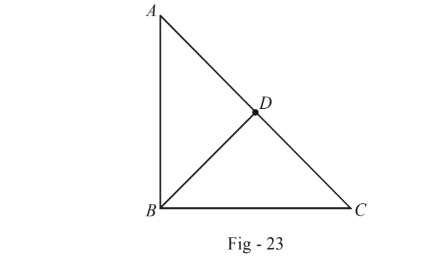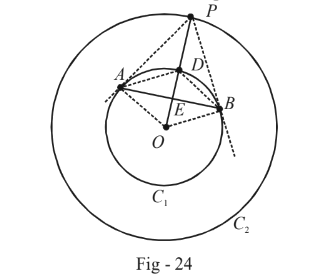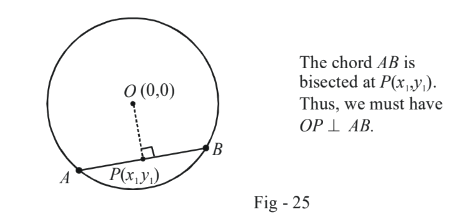# Examples On Tangents To Circles Set-3

Go back to  'Circles'

Example - 23

DIRECTOR CIRCLE

A point $$P$$ moves in such a way so that the tangents drawn from it to the circle $${x^2} + {y^2} = {a^2}$$ are perpendicular. Find the locus of $$P$$.

Solution: To contrast between the various alternatives available to us, we will use all of them here:From the figure, it is apparent that $$OTPS$$ is a square since all the angles are right angles and  $$OS = OT = a$$ .

Thus, $$\,OP = \sqrt 2 \,a$$ , i.e., the distance of $$P$$ from $$O$$ is always $$\sqrt 2 \,a,$$ i.e., $$P$$ lies on a circle of radius $$\sqrt 2 \,a$$. Thus, $$P$$ satisfies the equation

$\fbox{\begin{array}{*{20}{c}} {{x^2} + {y^2} = 2{a^2}}\end{array}}$

This circle is called the Director circle of the given circle.

### A CO-ORDINATE APPROACH - I

Let $$P$$ be the point $$(h,k).$$ The equation of the pair of tangents drawn from $$P$$ to the circle is

\begin{align}\,\,\,\,\,\,\,\,\,\,\,\,\,\,\,\,\,&{T^2} = S{S_1}\\ \Rightarrow \qquad &{(hx + ky - {a^2})^2} = ({x^2} + {y^2} - {a^2})({h^2} + {k^2} - {a^2})\end{align}

This combined equation will represent a pair of perpendicular straight lines if

\begin{align}\,\,\,\,\,\,\,\,\,\,\,\,&{\rm{Coeff}}{\rm{. of }}{x^2} + {\rm{Coeff}}{\rm{. of }}{y^2} = 0\\ \Rightarrow \qquad &({k^2} - {a^2}) + ({h^2} - {a^2}) = 0\\ \Rightarrow \qquad & {h^2} + {k^2} = 2{a^2}\end{align}

Using $$(x,y)$$ instead of $$(h,k)$$, we obtain the locus of $$P$$ in conventional form:

\begin{align}{{x^2} + {y^2} = 2{a^2}}\end{align}

### A CO-ORDINATE APPROACH - II

The equation of any tangent to the circle $${x^2} + {y^2} = {a^2}$$ can be written as $$y = mx + a\sqrt {1 + {m^2}} .$$

If this line passes through $$P(h,k),$$ the co-ordinates of $$P$$ must satisfy this equation:

\begin{align}\,\,\,\,\,\,\,\,\,\,\,\,\,\,\,\,&k = mh + a\sqrt {1 + {m^2}} \\\\ \Rightarrow \qquad & {(k - mh)^2} = {a^2}(1 + {m^2})\\\\ \Rightarrow \qquad & ({h^2} - {a^2}){m^2} - 2mhk + ({k^2} - {a^2}) = 0\end{align}

This is a quadratic in $$m$$ and will yield two values, say $${m_1}$$ and $${m_2},$$ which physically corresponds to the fact that two tangents can be drawn from $$P(h,k)$$ to the circle.

The two tangents are at a right angle if $${m_1}{m_2} = - 1$$

\begin{align}{} \Rightarrow\quad & \frac{{{k^2} - {a^2}}}{{{h^2} - {a^2}}} = - 1\\ \Rightarrow \quad & {h^2} + {k^2} = 2{a^2}\end{align}

Using $$(x,y)$$ instead of  $$k(h,k)$$ we obtain the locus of $$P$$:

$x^2 + y^2 = 2{a^2}$

If this question were to be encountered in an exam, the pure-geometric approach would certainly turn out to be the fastest !

Example - 24

$${C_1}$$ and $${C_2}$$ are two concentric circles, the radius of $${C_2}$$ being twice that of $${C_1}$$. From a point $$P$$  on $${C_2},$$ tangents $$PA$$ and $$PB$$ are drawn to $${C_1}.$$ Prove that the centroid of $$\Delta PAB$$ is on $${C_1}.$$

Solution: Let us again attempt this question using both a pure-geometric and a co-ordinate approach.

### Pure-geometric approach:

Recall the following straightforward theorem pertaining to right-angle triangles.$$\Delta$$ $$ABC$$ is right-angled at $$B$$ and $$BD$$ is the median drawn from $$B$$ to the opposite side $$AC$$. Then our theorem tells us that

$BD=AD=CD$

This can be proved using simple geometry

We will put this theorem to use in the current example.Since the radius $$r_2$$ of $$C_2$$  is twice that of $$C_1$$ ($$r_1$$ ) we have

\begin{align} &PO = 2\;OD\\ \Rightarrow \qquad & OD = PD & & & ...(1) \end{align}

Thus,$$D$$ is the mid-point of $$PO$$. This means that in $$\Delta OAP$$, $$AD$$ is the median to $$OP$$. By the theorem mentioned above, we have

$AD = OD = PD = {r_1}$

Thus, in quadrilateral $$ADBO,$$ we have $$AD = BD = OA = OB = {r_1}.$$ In other words, $$ADBO$$  is a parallelogram so $$E$$ is the midpoint of  $$OD.$$ Thus, \begin{align}ED =\frac {r_1}{2} = \frac{1}{2}OD\end{align}                ...(2)

Also, since  $$AE = EB,$$ $$PE$$  is the median of $$\Delta PAB.$$ Thus the centroid lies on $$PE.$$

From (1) and (2), we finally obtain $$PD = 2ED.$$  Thus, $$D$$ divides the median $$PE$$ in the ratio $$2 : 1$$ implying $$D$$ is the centroid which lies on $${C_1}.$$

The descriptive nature of the pure-geometric solution just provided might make it appear to be very long but actually only a few simple elementary geometry facts have been used.

### Co-ordinate approach

There’s no loss of generality in assuming that the two circles are centred at the origin. Thus, we can write their equations as

\begin{align} {{C_1}\,\,\,:}&{{x^2} + {y^2} = {r^2}}\\ {{C_2}\,\,\,:}&{{x^2} + {y^2} = 4{r^2}} \end{align}

Assume the point $$P$$ to have the co-ordinates $$(h,k).$$ The equation of $$AB$$ (the chord of contact) can then be written  as

${\rm{Equation}}\,{\rm{of}}\;AB:\,\,\,hx + ky = {a^2}$

We can evaluate the co-ordinates of $$A({x_1},{y_1})$$ and $$B({x_2},{y_2})$$ by simultaneously solving the equations for $${C_1}$$ and $$AB$$.

Thus, $${x_1}$$ and $${x_2}$$ will be the roots of

\begin{align}{}\,\,\,\,\,\,\,\,\,\,\,\,\,\,\,\,\,\,&{x^2} + {\left( {\frac{{{r^2} - hx}}{k}} \right)^2} = {r^2}\\\\ \Rightarrow \qquad &({h^2} + {k^2}){x^2} - 2{r^2}hx + {r^2}({r^2} - {k^2}) = 0\\\Rightarrow \qquad & {x_1} + {x_2} = \frac{{2{r^2}h}}{{{h^2} + {k^2}}}\;\; and \;\;{x_1}{x_2} = \frac{{{r^2}({r^2} - {k^2})}}{{{h^2} + {k^2}}} \qquad \qquad ... (3) \end{align}

$${y_1}$$ and $${y_2}$$ will be the roots of

\begin{align}{}\,\,\,\,\,\,\,\,\,\,\,\,\,\,\,\,\,\,&{\left( {\frac{{{r^2} - ky}}{h}} \right)^2} + {y^2} = {r^2}\\\\ \Rightarrow\qquad &({h^2} + {k^2}){y^2} - 2{r^2}ky + {r^2}({r^2} - {h^2}) = 0\\ \Rightarrow \qquad & {y_1} + {y_2} = \frac{{2{r^2}k}}{{{h^2} + {k^2}}}\;\; and \;\;{y_1}{y_2} = \frac{{{r^2}({r^2} - {h^2})}}{{{h^2} + {k^2}}} \qquad \qquad ... (4)\end{align}

If we let $$(t,s)$$ be the co-ordinates of the centroid $$G$$ of $$\Delta PAB,$$ we have

\left. \begin{align}{l}t = \frac{{{x_1} + {x_2} + h}}{3} & & \Rightarrow & 3t = h\left( {1 + \frac{{2{r^2}}}{{{h^2} + {k^2}}}} \right)\\s = \frac{{{y_1} + {y_2} + k}}{3} & & \Rightarrow & 3s = k\left( {1 + \frac{{2{r^2}}}{{{h^2} + {k^2}}}} \right)\end{align} \right\}\,\,\,\,{\rm{Using}}\left( 3 \right){\rm{and}}\left( 4 \right)

Finally, observe that

\begin{align}9({t^2} + {s^2}) = ({h^2} + {k^2}){\left( {1 + \frac{{2{r^2}}}{{{h^2} + {k^2}}}} \right)^2}\end{align}

But since $$(h,k)$$ lies on $${C_2},$$ we have $${h^2} + {k^2} = 4{r^2}.$$

Thus,

\begin{align} \,\,\,\,\,\,\,\,\,&9\left( {{t^2} + {s^2}} \right) = \left( {4{r^2}} \right)\left( {\frac{9}{4}} \right) = 9{r^2}\\ \Rightarrow \qquad & {t^2} + {s^2} = {r^2} \end{align}

implying that $$G(t,s)$$ lies on $${C_1}.$$

Since now you are in a good position to compare the two approaches, which one could you have rather chosen for this question, the pure-geometric one or the co-ordinate one!

Example - 25

Consider the circle $${x^2} + {y^2} = {a^2}.$$  A chord of this circle is bisected at the point $$P({x_1},{y_1}).$$ What is the equation of this chord ?

Solution: Convince yourself that such a chord will be unique, since it must be perpendicular to the line joining the origin to $$P({x_1},{y_1}),$$ as is clear from the figure below:The slope of $$AB$$ then becomes \begin{align}\frac{{ - {x_1}}}{{{y_1}}}\end{align} so its equation can be written simply as

\begin{align}{}\,\,\,\,\,\,\,\,\,\,\,\,\,\,\,\,&y - {y_1} = - \frac{{{x_1}}}{{{y_1}}}(x - {x_1})\\ \Rightarrow \qquad & x{x_1} + y{y_1} = x_1^{\,\,2} + y_1^{\,\,2}\end{align}

To make this equation look “better”, we subtract $${a^2}$$ from both sides

$x{x_1} + y{y_1} - {a^2} = x_1^{\,\,\,2} + y_1^{\,\,\,2} - {a^2}$

so that it can now be  written concisely as

$\fbox{\begin{array}{*{20}{c}} {T({x_1},{y_1}) = S({x_1},{y_1})} \end{array}}$

Of course, this is easily generalised to the case when the equation of the circle is in the general form $${x^2} + {y^2} + 2gx + 2fy + c = 0;$$ the result obtained is the same. The next example discusses a good application of this concept.